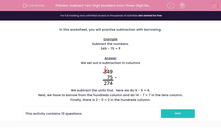# Subtract Two-Digit Numbers from Three-Digit Numbers

In this worksheet, students subtract two-digit numbers from three-digit numbers using a written column method.This content is premium and exclusive to EdPlace subscribers.Key stage:  KS 2

Curriculum topic:   Maths and Numerical Reasoning

Curriculum subtopic:   Mixed Problems

Difficulty level:#### Worksheet Overview

In this worksheet, you will practise subtraction with borrowing.

Example

Subtract the numbers.

349 - 75 = ?

We set out a subtraction in columnsWe subtract the units first.  Here we do 9 - 5 = 4.

Next, we have to borrow from the hundreds column and do 14 - 7 = 7 in the tens column.

Finally, there is 2 - 0 = 2 in the hundreds column.

### What is EdPlace?

We're your National Curriculum aligned online education content provider helping each child succeed in English, maths and science from year 1 to GCSE. With an EdPlace account you’ll be able to track and measure progress, helping each child achieve their best. We build confidence and attainment by personalising each child’s learning at a level that suits them.

Get started